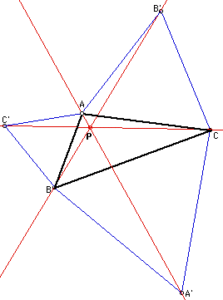# What is This Point?Take an arbitrary triangle ABC. On its three sides, construct the equilateral triangles ABC’, ACB’, and BCA’. Then construct lines AA’, BB’ and CC’. What do you observe?

What is special about the point of intersection, P, of the three lines?

Can you write a story problem about the above construction?Extension:
Amy thinks that point P represents the location in the triangle that has the smallest sum of distances to the vertices of the triangles. Do you think Amy’s claim is true? Why or Why not?

Construct circumcircles of the equilateral triangles ABC’, ACB’, and BCA’. What do you observe?